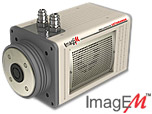•Main Site
•Events
•Support
•Corporate
•News

# Featured ArticleElectron Multiplying Charge-Coupled Devices (EMCCDs)

By incorporating on-chip multiplication gain, the electron multiplying CCD achieves, in an all solid-state sensor, the single-photon detection sensitivity typical of intensified or electron-bombarded CCDs at much lower cost and without compromising the quantum efficiency and resolution characteristics of the conventional CCD structure.

# Interactive Flash Tutorials

### Conversion Efficiency

Conversion efficiency is one way of describing the relationship between gray levels in software and the related signal levels in an image. The term describes the number of electrons in a CCD that are used to equal an intensity change of one gray level in the software.

Other terms used are "ADU" (analog to digital units) and "Counts" (electron counts per gray level) but all mean the same thing.

As described in the CCD tutorial, silicon based detectors like CCDs and CMOS detectors convert the energy from a photon into an electronic signal by displacing an electron within a pixel and leaving behind a "hole pair". The relationship of photons to electrons (or hole pairs) is 1 to 1 so each electron in the pixel represents one detected photon. As the number of electrons in a pixel increases, different signal levels become possible. The number of possible signal levels in a camera is determined by the digitizer which divides the maximum signal level (the Full Well Capacity of a pixel) into "bits", each of which has an equal number of electrons. Each bit is represented in the software as a gray level.

The relationship between the number of bits and the maximum signal level is the conversion efficiency. In digital cameras there is an additional factor, the "digitizer offset" that must be subtracted from the number of digitizer bits to make the conversion accurately.

Example: Camera with 20,000 electrons FWC, 12 bit digitizer (4095 bits or gray levels), and 100 bits digitizer offset.

##### Conversion Efficiency = 20,000e / (4096bits - 100bits) = 5e/bit

The most important reason to understand conversion efficiency is it provides the signal information in real quantitative numbers, signal electrons, rather than arbitrary gray level values.